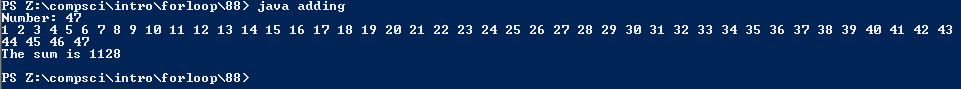# Assignemnt #88: Adding Values with a For Loop

## Code

```    /// Name: Cassie
/// Period: 6
/// Program Name: Adding Value with a For Loop
/// Date Finished: 12/15/2015

import java.util.Scanner;

public static void main( String[] args ) {

Scanner keyboard = new Scanner(System.in);

System.out.print( "Number: " );
int number = keyboard.nextInt();
int sum = 0;

for ( int n = 1; n <= number; n = n+1 ) {
System.out.print( n + " " );

sum = sum + n;
}

System.out.println();
System.out.println( "The sum is " + sum );
System.out.println();

}
}
```

### Picture of the output